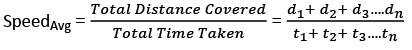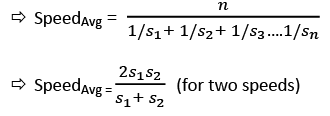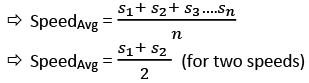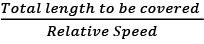# Important Formulae: Speed, Time & Distance Notes | Study Quantitative Aptitude (Quant) - CAT

## CAT: Important Formulae: Speed, Time & Distance Notes | Study Quantitative Aptitude (Quant) - CAT

The document Important Formulae: Speed, Time & Distance Notes | Study Quantitative Aptitude (Quant) - CAT is a part of the CAT Course Quantitative Aptitude (Quant).
All you need of CAT at this link: CAT

Time Speed and Distance

Speed = Distance / Time
1 kmph = 5/18 m/sec; 1 m/sec = 18/5 kmphIf the distance covered is constant then the average speed is the Harmonic Mean of the values (s1, s2, s3….sn)If the time taken is constant then the average speed is the Arithmetic Mean of the values (s1, s2, s3….sn)EduRev's Tip: Given that the distance between two points is constant, then
⇒ If the speeds are in Arithmetic Progression, then the times taken are in Harmonic Progression
⇒ If the speeds are in Harmonic Progression, then the times taken are in Arithmetic Progression
For Trains, time taken =For Boats,
SpeedUpstream = SpeedBoat – SpeedRiver
SpeedDownstream = SpeedBoat + SpeedRiver
SpeedBoat = (SpeedDownstream + SpeedUpstream) / 2
SpeedRiver = (SpeedDownstream – SpeedUpstream) / 2
For Escalators, The difference between escalator problems and boat problems is that escalator can go either up or down.

The document Important Formulae: Speed, Time & Distance Notes | Study Quantitative Aptitude (Quant) - CAT is a part of the CAT Course Quantitative Aptitude (Quant).
All you need of CAT at this link: CATUse Code STAYHOME200 and get INR 200 additional OFF

## Quantitative Aptitude (Quant)

155 videos|129 docs|131 tests

### How to Prepare for CAT

Read our guide to prepare for CAT which is created by Toppers & the best Teachers

Track your progress, build streaks, highlight & save important lessons and more!

,

,

,

,

,

,

,

,

,

,

,

,

,

,

,

,

,

,

,

,

,

,

,

,

;